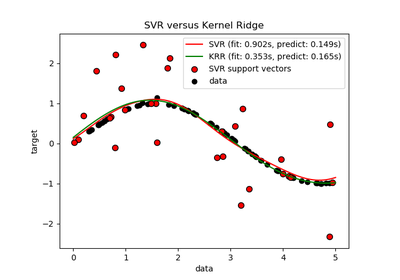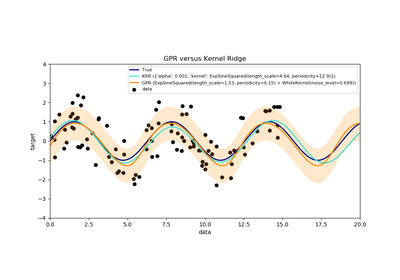# `sklearn.kernel_ridge`.KernelRidge¶

class `sklearn.kernel_ridge.``KernelRidge`(alpha=1, kernel=’linear’, gamma=None, degree=3, coef0=1, kernel_params=None)[source]

Kernel ridge regression.

Kernel ridge regression (KRR) combines ridge regression (linear least squares with l2-norm regularization) with the kernel trick. It thus learns a linear function in the space induced by the respective kernel and the data. For non-linear kernels, this corresponds to a non-linear function in the original space.

The form of the model learned by KRR is identical to support vector regression (SVR). However, different loss functions are used: KRR uses squared error loss while support vector regression uses epsilon-insensitive loss, both combined with l2 regularization. In contrast to SVR, fitting a KRR model can be done in closed-form and is typically faster for medium-sized datasets. On the other hand, the learned model is non-sparse and thus slower than SVR, which learns a sparse model for epsilon > 0, at prediction-time.

This estimator has built-in support for multi-variate regression (i.e., when y is a 2d-array of shape [n_samples, n_targets]).

Read more in the User Guide.

Parameters: alpha : {float, array-like}, shape = [n_targets] Small positive values of alpha improve the conditioning of the problem and reduce the variance of the estimates. Alpha corresponds to `(2*C)^-1` in other linear models such as LogisticRegression or LinearSVC. If an array is passed, penalties are assumed to be specific to the targets. Hence they must correspond in number. kernel : string or callable, default=”linear” Kernel mapping used internally. A callable should accept two arguments and the keyword arguments passed to this object as kernel_params, and should return a floating point number. gamma : float, default=None Gamma parameter for the RBF, laplacian, polynomial, exponential chi2 and sigmoid kernels. Interpretation of the default value is left to the kernel; see the documentation for sklearn.metrics.pairwise. Ignored by other kernels. degree : float, default=3 Degree of the polynomial kernel. Ignored by other kernels. coef0 : float, default=1 Zero coefficient for polynomial and sigmoid kernels. Ignored by other kernels. kernel_params : mapping of string to any, optional Additional parameters (keyword arguments) for kernel function passed as callable object. dual_coef_ : array, shape = [n_samples] or [n_samples, n_targets] Representation of weight vector(s) in kernel space X_fit_ : {array-like, sparse matrix}, shape = [n_samples, n_features] Training data, which is also required for prediction

`Ridge`
Linear ridge regression.
`SVR`
Support Vector Regression implemented using libsvm.

References

• Kevin P. Murphy “Machine Learning: A Probabilistic Perspective”, The MIT Press chapter 14.4.3, pp. 492-493

Examples

```>>> from sklearn.kernel_ridge import KernelRidge
>>> import numpy as np
>>> n_samples, n_features = 10, 5
>>> rng = np.random.RandomState(0)
>>> y = rng.randn(n_samples)
>>> X = rng.randn(n_samples, n_features)
>>> clf = KernelRidge(alpha=1.0)
>>> clf.fit(X, y)
KernelRidge(alpha=1.0, coef0=1, degree=3, gamma=None, kernel='linear',
kernel_params=None)
```

Methods

 `fit`(X[, y, sample_weight]) Fit Kernel Ridge regression model `get_params`([deep]) Get parameters for this estimator. `predict`(X) Predict using the kernel ridge model `score`(X, y[, sample_weight]) Returns the coefficient of determination R^2 of the prediction. `set_params`(**params) Set the parameters of this estimator.
`__init__`(alpha=1, kernel=’linear’, gamma=None, degree=3, coef0=1, kernel_params=None)[source]
`fit`(X, y=None, sample_weight=None)[source]

Fit Kernel Ridge regression model

Parameters: X : {array-like, sparse matrix}, shape = [n_samples, n_features] Training data y : array-like, shape = [n_samples] or [n_samples, n_targets] Target values sample_weight : float or array-like of shape [n_samples] Individual weights for each sample, ignored if None is passed. self : returns an instance of self.
`get_params`(deep=True)[source]

Get parameters for this estimator.

Parameters: deep : boolean, optional If True, will return the parameters for this estimator and contained subobjects that are estimators. params : mapping of string to any Parameter names mapped to their values.
`predict`(X)[source]

Predict using the kernel ridge model

Parameters: X : {array-like, sparse matrix}, shape = [n_samples, n_features] Samples. C : array, shape = [n_samples] or [n_samples, n_targets] Returns predicted values.
`score`(X, y, sample_weight=None)[source]

Returns the coefficient of determination R^2 of the prediction.

The coefficient R^2 is defined as (1 - u/v), where u is the residual sum of squares ((y_true - y_pred) ** 2).sum() and v is the total sum of squares ((y_true - y_true.mean()) ** 2).sum(). The best possible score is 1.0 and it can be negative (because the model can be arbitrarily worse). A constant model that always predicts the expected value of y, disregarding the input features, would get a R^2 score of 0.0.

Parameters: X : array-like, shape = (n_samples, n_features) Test samples. y : array-like, shape = (n_samples) or (n_samples, n_outputs) True values for X. sample_weight : array-like, shape = [n_samples], optional Sample weights. score : float R^2 of self.predict(X) wrt. y.
`set_params`(**params)[source]

Set the parameters of this estimator.

The method works on simple estimators as well as on nested objects (such as pipelines). The latter have parameters of the form `<component>__<parameter>` so that it’s possible to update each component of a nested object.

Returns: self :

## Examples using `sklearn.kernel_ridge.KernelRidge`¶Comparison of kernel ridge regression and SVRComparison of kernel ridge and Gaussian process regression